# KR20120030813A - Method of processing data and display apparatus performing the same - Google Patents

Method of processing data and display apparatus performing the same Download PDF

## Info

Publication number
KR20120030813A
KR20120030813A KR1020100092575A KR20100092575A KR20120030813A KR 20120030813 A KR20120030813 A KR 20120030813A KR 1020100092575 A KR1020100092575 A KR 1020100092575A KR 20100092575 A KR20100092575 A KR 20100092575A KR 20120030813 A KR20120030813 A KR 20120030813A
Authority
KR
South Korea
Prior art keywords
frame
motion
original image
interpolation
image frames
Prior art date
Application number
KR1020100092575A
Other languages
Korean (ko)
Inventor
김경우
박동준
이영재
Original Assignee
삼성전자주식회사
Priority date (The priority date is an assumption and is not a legal conclusion. Google has not performed a legal analysis and makes no representation as to the accuracy of the date listed.)
Filing date
Publication date
Application filed by 삼성전자주식회사 filed Critical 삼성전자주식회사
Priority to KR1020100092575A priority Critical patent/KR20120030813A/en
Publication of KR20120030813A publication Critical patent/KR20120030813A/en

• 230000020169 heat generation Effects 0 abstract 1
• 239000000203 mixtures Substances 0 abstract 1
• 238000003672 processing method Methods 0 abstract 1

## Images

•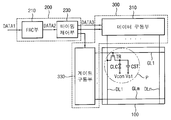•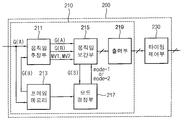•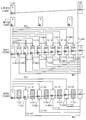•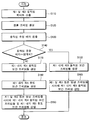•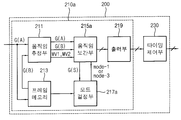••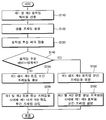•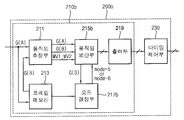•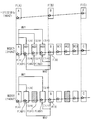•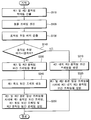•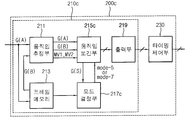•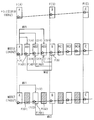•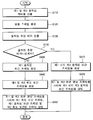•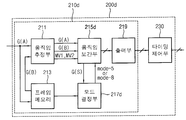•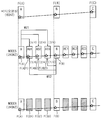•## Classifications

• GPHYSICS
• G09EDUCATION; CRYPTOGRAPHY; DISPLAY; ADVERTISING; SEALS
• G09GARRANGEMENTS OR CIRCUITS FOR CONTROL OF INDICATING DEVICES USING STATIC MEANS TO PRESENT VARIABLE INFORMATION
• G09G3/00Control arrangements or circuits, of interest only in connection with visual indicators other than cathode-ray tubes
• G09G3/20Control arrangements or circuits, of interest only in connection with visual indicators other than cathode-ray tubes for presentation of an assembly of a number of characters, e.g. a page, by composing the assembly by combination of individual elements arranged in a matrix no fixed position being assigned to or needed to be assigned to the individual characters or partial characters
• GPHYSICS
• G09EDUCATION; CRYPTOGRAPHY; DISPLAY; ADVERTISING; SEALS
• G09GARRANGEMENTS OR CIRCUITS FOR CONTROL OF INDICATING DEVICES USING STATIC MEANS TO PRESENT VARIABLE INFORMATION
• G09G3/00Control arrangements or circuits, of interest only in connection with visual indicators other than cathode-ray tubes
• G09G3/20Control arrangements or circuits, of interest only in connection with visual indicators other than cathode-ray tubes for presentation of an assembly of a number of characters, e.g. a page, by composing the assembly by combination of individual elements arranged in a matrix no fixed position being assigned to or needed to be assigned to the individual characters or partial characters
• G09G3/34Control arrangements or circuits, of interest only in connection with visual indicators other than cathode-ray tubes for presentation of an assembly of a number of characters, e.g. a page, by composing the assembly by combination of individual elements arranged in a matrix no fixed position being assigned to or needed to be assigned to the individual characters or partial characters by control of light from an independent source
• G09G3/36Control arrangements or circuits, of interest only in connection with visual indicators other than cathode-ray tubes for presentation of an assembly of a number of characters, e.g. a page, by composing the assembly by combination of individual elements arranged in a matrix no fixed position being assigned to or needed to be assigned to the individual characters or partial characters by control of light from an independent source using liquid crystals
• G09G3/3611Control of matrices with row and column drivers
• G09G3/3648Control of matrices with row and column drivers using an active matrix
• GPHYSICS
• G09EDUCATION; CRYPTOGRAPHY; DISPLAY; ADVERTISING; SEALS
• G09GARRANGEMENTS OR CIRCUITS FOR CONTROL OF INDICATING DEVICES USING STATIC MEANS TO PRESENT VARIABLE INFORMATION
• G09G5/00Control arrangements or circuits for visual indicators common to cathode-ray tube indicators and other visual indicators
• HELECTRICITY
• H04ELECTRIC COMMUNICATION TECHNIQUE
• H04NPICTORIAL COMMUNICATION, e.g. TELEVISION
• H04N19/00Methods or arrangements for coding, decoding, compressing or decompressing digital video signals
• H04N19/50Methods or arrangements for coding, decoding, compressing or decompressing digital video signals using predictive coding
• H04N19/59Methods or arrangements for coding, decoding, compressing or decompressing digital video signals using predictive coding involving spatial sub-sampling or interpolation, e.g. alteration of picture size or resolution
• HELECTRICITY
• H04ELECTRIC COMMUNICATION TECHNIQUE
• H04NPICTORIAL COMMUNICATION, e.g. TELEVISION
• H04N7/00Television systems
• H04N7/01Conversion of standards, e.g. involving analogue television standards or digital television standards processed at pixel level
• H04N7/0135Conversion of standards, e.g. involving analogue television standards or digital television standards processed at pixel level involving interpolation processes
• H04N7/014Conversion of standards, e.g. involving analogue television standards or digital television standards processed at pixel level involving interpolation processes involving the use of motion vectors
• GPHYSICS
• G09EDUCATION; CRYPTOGRAPHY; DISPLAY; ADVERTISING; SEALS
• G09GARRANGEMENTS OR CIRCUITS FOR CONTROL OF INDICATING DEVICES USING STATIC MEANS TO PRESENT VARIABLE INFORMATION
• G09G2320/00Control of display operating conditions
• G09G2320/02Improving the quality of display appearance
• G09G2320/0233Improving the luminance or brightness uniformity across the screen

## Abstract

PURPOSE: An image data processing method and a display apparatus using the same are provided to insert a brightness interpolation frame or a mixture of a movement interpolation frame and the brightness interpolation frame, thereby solving heat generation and image shaking problems. CONSTITUTION: A data processing part(200) comprises a movement estimation part(211), a movement interpolation part(215), and an output unit(219). The movement estimation part outputs a first movement vector and a second movement vector. The movement interpolation part generates a sample frame and a brightness interpolation frame. The output part inserts the brightness interpolation frame between first and second original image frames. A panel driving part outputs data of the frames on a display panel.

## Description

Image data processing method and display device performing the same {METHOD OF PROCESSING DATA AND DISPLAY APPARATUS PERFORMING THE SAME}

The present invention relates to an image data processing method and a display device for performing the same, and more particularly, to an image data processing method for displaying an image of a high frame rate and a display device for performing the same.

In general, the liquid crystal display includes a liquid crystal display panel displaying an image using light transmittance of liquid crystal, and a backlight assembly disposed under the liquid crystal display panel to provide light to the liquid crystal display panel.

The liquid crystal display (LCD) has been expanding the market while improving the performance of text and still image display as a monitor such as a notebook computer or a PC. Recently, it has been employed in application technologies such as computer games using high-definition video and three-dimensional stereoscopic images.

In general, a frame rate control technique is used to increase the frame rate of a signal having a frequency of 60 Hz to a high frequency of 120 Hz or 240 Hz to realize a high resolution video. To this end, a motion interpolation frame in which motion is interpolated is generated by motion estimation and motion interpolation, and the motion interpolation frame is inserted between a current frame and a previous frame. In order to insert the motion interpolation frame, a high speed frame driving method is basically used.

As described above, due to many operations for motion estimation and interpolation during high-speed frame driving, a heat generation problem occurs in a chip generating the motion interpolation frame. In addition, when a motion estimation error occurs, a problem may occur in which an image is not smooth and the image is shaken or shaken due to the inserted motion interpolation frame.

Accordingly, the technical problem of the present invention was conceived in this respect, and an object of the present invention is to provide a video data processing method for improving display quality.

Another object of the present invention is to provide a display device suitable for performing the image data processing method.

In the image data processing method according to an embodiment for realizing the above object of the present invention, the first and the second image by motion estimation of the data of the first original image frame and the second original image frame different from the first original image frame Calculate two motion vectors. A sample frame is generated using the first and second motion vectors. At least one luminance interpolation frame having an average luminance value of two adjacent image frames is generated according to a result of the comparison between the first original image frame and the sample frame. The luminance interpolation frame is inserted between the first and second original image frames.

In an embodiment of the present invention, the generating of the luminance interpolation frame may include using two adjacent image frames when a motion estimation error exceeds a preset threshold as a result of comparing the first original image frame with the sample frame. Generate the luminance interpolation frame.

In an embodiment of the present invention, the two adjacent image frames may be the first and second original image frames.

In an embodiment of the present invention, the first and second original image frames may be received at a frame frequency of 60 Hz, and the first and second original image frames into which the luminance interpolation frame is inserted may be output at a frame frequency of 240 Hz. have.

In another embodiment of the present invention, the first and second original image frames are received at a frame frequency of 24 Hz, and the first and second original image frames into which the luminance interpolation frame is inserted are output at a frame frequency of 240 Hz. Can be.

In an embodiment of the present invention, generating at least one motion interpolation frame in consideration of weights of the first and second motion vectors and inserting the motion interpolation frame between the first and second original frames. Wherein the two adjacent image frames are at least one of the first original image frame and the motion interpolation frame, the adjacent motion interpolation frames, and the motion interpolation frames and the second original image frames. Can be.

In an embodiment of the present invention, the motion interpolation frame and the luminance interpolation frame may be inserted at a ratio of 1: 2 or 2: 1.

In an embodiment of the present invention, the motion interpolation frame and the luminance interpolation frame may be inserted at a ratio of 4: 5.

According to another exemplary embodiment of the present invention, a display device may include a display panel, a data processor, and a panel driver. The display panel displays an image. The data processor generates a sample frame by using first and second motion vectors calculated by motion estimation of data of a first original image frame and a second original image frame different from the first original image frame. Generate at least one luminance interpolation frame having an average luminance value of two adjacent image frames according to a comparison result of the original image frame and the sample frame, and between the first and second original image frames, Insert and print The panel driver outputs data of frames output from the data processor to the display panel.

In an embodiment of the present invention, the data processor may include a motion estimator for calculating the first and second motion vectors, a motion interpolator for generating the sample frame and the luminance interpolation frame, and the first and second original images. It may include an output unit for inserting and outputting the luminance interpolation frame between the frames.

According to such an image data processing method and a display device which performs the same, a luminance interpolation frame having an average luminance value of adjacent frames when a motion estimation error occurs, or a mixture of the luminance interpolation frame and the motion interpolation frame is inserted and inserted, such as a heating problem and image shaking. You can solve the picture quality problem. Therefore, display quality can be improved.

1 is a block diagram of a display device according to a first exemplary embodiment of the present invention.
FIG. 2 is a block diagram of the data processor shown in FIG. 1.
FIG. 3 is a conceptual diagram illustrating a motion interpolation method of the motion interpolator shown in FIG. 2.
4 is a flowchart for describing a method of driving a data processor illustrated in FIG. 2.
5 is a block diagram of a data processing unit according to Embodiment 2 of the present invention.
FIG. 6 is a conceptual diagram illustrating a motion interpolation method of the motion interpolator shown in FIG. 5.
FIG. 7 is a flowchart for describing a method of driving the data processor illustrated in FIG. 5.
8 is a block diagram of a data processing unit according to Embodiment 3 of the present invention.
FIG. 9 is a conceptual diagram illustrating a motion interpolation method of the motion interpolator shown in FIG. 8.
FIG. 10 is a flowchart for describing a driving method of the data processor illustrated in FIG. 8.
11 is a block diagram of a data processing unit according to Embodiment 4 of the present invention.
FIG. 12 is a conceptual diagram illustrating a motion interpolation method of the motion interpolator shown in FIG. 11.
FIG. 13 is a flowchart for describing a method of driving a data processor illustrated in FIG. 11.
14 is a block diagram of a data processing unit according to Embodiment 5 of the present invention.
FIG. 15 is a conceptual diagram illustrating a motion interpolation method of the motion interpolator shown in FIG. 14.
16 is a flowchart for describing a method of driving the data processor illustrated in FIG. 14.

Hereinafter, with reference to the drawings will be described in detail preferred embodiments of the present invention.

Example 1

1 is a block diagram of a display device according to a first exemplary embodiment of the present invention.

Referring to FIG. 1, a display panel 100, a data processor 200, and a panel driver 300 according to an exemplary embodiment are included.

The display panel 100 includes a plurality of gate lines GL1 to GLm, a plurality of data lines DL1 to DLn, and a plurality of pixels P. Each pixel P includes a driving element TR, a liquid crystal capacitor CLC and a storage capacitor CST electrically connected to the driving element TR. The display panel 100 may include two substrates facing each other and a liquid crystal layer interposed between the two substrates.

The data processor 200 includes a frame rate control (FRC) unit 210 and a timing controller 230.

The FRC unit 210 converts the frame data DATA1 of the input image input from the outside at the first frame frequency into a second frame frequency faster than the first frame frequency. The first frame frequency may be 60 Hz and the second frame frequency may be 240 Hz. The FRC unit 210 generates a sample frame using first and second motion vectors calculated by motion estimation of data of a first original video frame and a second original video frame different from the first original video frame. The FRC unit 210 generates at least one luminance interpolation frame having an average luminance value of two image frames adjacent to a comparison result between the first original image frame and the sample frame. The FRC unit 210 may insert the rear interpolation frame between the first and second image frames to convert the frame rate of the input image.

The timing controller 230 receives frame rate converted data DATA2 from the data processor 200 and outputs the data DATA2 to the panel driver 300 in units of horizontal lines. In addition, the timing controller 230 generates a control signal for controlling the driving timing of the panel driver 300 using a control signal received from the outside.

The panel driver 300 may include a data driver 310 and a gate driver 330.

The data driver 310 converts the data DATA3 received from the timing controller 230 into an analog data voltage. The data driver 310 outputs the data voltages to the data lines DL1 to DLn.

The gate driver 330 outputs gate signals to the gate lines GL1 to GLm in synchronization with the output of the data driver 310.

FIG. 2 is a block diagram of the data processor shown in FIG. 1. 3 is a conceptual diagram for describing an operation of a motion interpolator illustrated in FIG. 2.

1 to 3, the data processor 200 includes the FRC unit 210 and the timing controller 230. The FRC unit 210 may include a motion estimator 211, a frame memory 213, a motion interpolator 215, a mode determiner 217, and an output unit 219.

The motion estimator 211 performs data G (A) of the first original image frame input from the outside and data G (B) of the second original image frames input from the frame memory 213. The first and second motion vectors MV1 and MV2 are calculated by estimating. Here, the first motion vector MV1 is a motion vector calculated based on the change of the second original video frame F (B) based on the first original video frame F (A). The second motion vector MV2 is a motion vector calculated based on the change of the first original video frame F (A) based on the second original video frame F (B). The first and second motion vectors MV1 and MV2 have the same size but different directions. The motion estimator 211 may estimate motion in units of blocks by using a block matching algorithm (BMA). In addition, the motion estimator 211 may estimate motion in units of pixels using a pixel recursive algorithm (PRA).

The motion interpolator 215 is operated in the first interpolation mode MODE 1 or the second interpolation mode MODE 2 under the control of the mode determiner 217. The first interpolation mode is a mode for generating a plurality of motion interpolation frames in consideration of weights of the first and second motion vectors MV1 and MV2, and the second interpolation mode MODE 2 is the motion interpolation frame. And luminance interpolation frames. The luminance interpolation frames have an average luminance value of two adjacent frames.

As shown in FIG. 3, when the input image is a film image, the film image has a frame frequency of 24 Hz. The film image is 3: 2 pulled down by an external controller (not shown) and converted to a frame frequency of 60 Hz. The motion interpolator 215 receives image frames converted to a frame frequency of 60 Hz. The 3: 2 pull-down is a method of generating five fields from two original image frames. For example, three fields are generated from the first original image frame and two fields are generated from the second original image frame. The FRC unit 210 may compare the received image frames and determine whether the image frames are a film image having a frame frequency of 24 Hz or a video image having a frame frequency of 60 Hz.

When the motion interpolator 215 receives the first interpolation mode signal mode-1 from the mode determiner 217, the motion interpolator 215 considers the weights of the first and second motion vectors MV1 and MV2. First, second, third, fourth, fifth, sixth, seventh, eighth and ninth motion interpolation frames F (AB1), F (AB2), F (AB3), and F (AB4). ), F (AB5), F (AB6), F (AB7), F (AB8), and F (AB9)).

For example, the first motion interpolation frame F (AB1) is applied with a weight of 1/10 for the first motion vector MV1 and 9/10 for the second motion vector MV2. Can be generated by applying a weight. In addition, the second motion interpolation frame F (AB2) applies a weight of 2/10 to the first motion vector MV1 and a weight of 8/10 to the second motion vector MV2. Can be generated by applying. The second motion interpolation frame F (AB2) applies a weight of 3/10 to the first motion vector MV1 and a weight of 7/10 to the second motion vector MV2. Can be generated. The fourth motion interpolation frame F (AB4) applies a weight of 4/10 to the first motion vector MV1 and a weight of 6/10 to the second motion vector MV2. Can be generated. The fifth motion interpolation frame F (AB5) applies a weight of 5/10 to the first motion vector MV1 and a weight of 5/10 to the second motion vector MV2. Can be generated. The sixth motion interpolation frame may be generated by applying a weight of 6/10 to the first motion vector MV1 and applying a weight of 4/10 to the second motion vector MV2. The seventh motion interpolation frame F (AB7) is applied with a weight of 7/10 for the first motion vector MV1 and a weight of 3/10 with respect to the second motion vector MV2. Can be generated. The eighth motion interpolation frame F (AB8) applies a weight of 8/10 to the first motion vector MV1 and a weight of 2/10 to the second motion vector MV2. Can be generated. The ninth motion interpolation frame F (AB9) is applied with a weight of 9/10 for the first motion vector MV1 and a weight of 1/10 with respect to the second motion vector MV2. Can be generated.

On the other hand, when receiving the signal from the mode determiner 217 in the second interpolation mode, the motion interpolator 215 is a first in consideration of the weight of the first and second motion vectors (MV1, MV2) Generate first, second, third and fourth motion interpolation frames F (AB1), F (AB2), F (AB3) and F (AB4). For example, the first motion interpolation frame F (AB1) is applied with a weight of 2/10 for the first motion vector MV1 and 8/10 for the second motion vector MV2. Can be generated by applying a weight. The second motion interpolation frame F (AB2) applies a weight of 4/10 to the first motion vector MV1 and a weight of 6/10 to the second motion vector MV2. Can be generated. The third motion interpolation frame F (AB3) applies a weight of 6/10 to the first motion vector MV1 and a weight of 4/10 to the second motion vector MV2. Can be generated. The fourth motion interpolation frame F (AB4) applies a weight of 8/10 to the first motion vector MV1 and a weight of 2/10 to the second motion vector MV2. Can be generated.

The motion interpolation unit 215 may include the first and second original image frames F (AB1) and F (AB2) and the first to fourth motion interpolation frames F (AB1) to F (AB4). Generate the first, second, third, fourth and fifth luminance interpolation frames F (G1), F (G2), F (G3), F (G4), and F (G5). do. The first to fifth luminance interpolation frames F (G1 to F (G5)) may each have an average luminance value of two adjacent frames.

For example, the first luminance interpolation frame F (G1) may have an average luminance value of the first original image frame F (A) and the first motion interpolation frame F (AB1). . The first luminance interpolation frame F (G1) is inserted between the first original image frame F (A) and the first motion interpolation frame F (AB1). The second luminance interpolation frame F (G2) may have an average luminance value of the first and second motion interpolation frames F (AB1) and F (AB2). The second luminance interpolation frame F (G2) is inserted between the first and second motion interpolation frames F (AB1) and F (AB2). The third luminance interpolation frame F (G3) may have an average luminance value of the second and third motion interpolation frames F (AB2) and F (AB3). The third luminance interpolation frame F (G3) is inserted between the second and third motion interpolation frames F (AB2) and F (AB3). The fourth luminance interpolation frame F (G4) may have an average luminance value of the third and fourth motion interpolation frames F (AB3) and F (AB4). The fourth luminance interpolation frame F (G4) is inserted between the third and fourth motion interpolation frames F (AB3) and F (AB4). The fifth luminance interpolation frame F (G5) may have an average luminance value of the fourth motion interpolation frame F (AB4) and the second original image frame F (B). The fifth luminance interpolation frame F (G5) is inserted between the fourth motion interpolation frame F (AB4) and the second original image frame F (B).

The mode determiner 217 detects a motion estimation error, and transmits the first interpolation mode signal (mode-1) to the motion interpolator 215 according to whether the motion estimation error exceeds a preset threshold. The second interpolation mode signal mode-2 may be output. The motion estimation error may be detected by comparing the data G (S) of the sample frame with the data G (A) of the first original image frame. Here, the sample frame may be the first motion interpolation frame F (AB1). The mode determiner 217 outputs the first interpolation mode signal mode-1 to the motion interpolator 215 when the motion estimation error is less than or equal to the threshold. In contrast, when the motion estimation error exceeds the threshold, the mode determiner 217 outputs the second interpolation mode signal mode-2 to the motion interpolator 215.

The output unit 219 may include the first to ninth motion interpolation frames F (AB1) to F (AB9) between the first and second original image frames F (A) and F (B). ) Or the first to fourth motion interpolation frames F (AB1 to F (AB4)) and the first to fifth luminance interpolation frames F (G1 to F (G9)). .

4 is a flowchart for describing a method of driving a data processor illustrated in FIG. 2.

2 to 4, when data of frames corresponding to the film image is received from the outside, the motion estimator 211 compares the data of the frames with data of the first and second original image frames. (G (A), G (B)) is detected.

The motion estimator 211 calculates the first and second motion vectors MV1 and MV2 by motion estimation of the data G (A) and G (B) of the first and second original image frames. (Step S110).

The motion interpolator 215 generates the sample frame in consideration of the weights of the first and second motion vectors MV1 and MV2 (step S120). The motion interpolator 215 outputs the data G (S) of the sample frame to the mode determiner 217.

The mode determiner 217 may receive the data G (S) of the sample frame received from the motion interpolator 215 and the data G (A (A) of the first original image frame stored in the frame memory 213. ) Is compared to detect a motion estimation error (step S130).

The mode determination unit 217 checks whether the motion estimation error exceeds the threshold (step S140). The mode determiner 217 outputs the first interpolation mode signal mode-1 to the motion interpolator 215 when the motion estimation error is less than or equal to the threshold.

The motion interpolation unit 215 receiving the first interpolation mode signal mode-1 applies the weights of the first and second motion vectors MV1 and MV2 to the first to ninth motion interpolation frames. Fields F (AB1) to F (AB9) are generated (step S150).

The output unit 219 may include the first to ninth motion interpolation frames F (AB1) to F (AB9) between the first and second original image frames F (A) and F (B). ) Is inserted and output to the timing controller 230 (step S160).

Meanwhile, the mode determiner 217 outputs the second interpolation mode signal mode-2 to the motion interpolator 215 when the motion estimation error exceeds the threshold.

The motion interpolation unit 215 receiving the second interpolation mode signal mode-2 may take into account the weights of the first and second motion vectors MV1 and MV2, and may include the first to fourth motion interpolation frames. Fields F (AB1) to F (AB4) are generated (step S170).

The motion interpolation unit 215 may include the first and second original image frames F (AB1) and F (AB2) and the first to fourth motion interpolation frames F (AB1) to F (AB4). Using the first to fifth luminance interpolation frames (F (G1) to F (G5)) to generate (S180).

The output unit 219 may include the first luminance interpolation frame F (G1) and the first motion interpolation frame between the first and second original image frames F (A) and F (B). F (AB1)), the second luminance interpolation frame F (G2), the second motion interpolation frame F (AB2), the third luminance interpolation frame F (G3), and the third motion interpolation The frame F (AB3), the fourth luminance interpolation frame F (G4), the fourth motion interpolation frame F (AB4) and the fifth luminance interpolation frame F (G5) are sequentially inserted. And outputs the result to the timing controller 230 (step S190).

According to the present embodiment, when the motion estimation error exceeds the threshold, the motion interpolation frame and the luminance interpolation frame are interposed between the first and second original image frames F (A) and F (B). Inserted at a ratio of 4: 5. Accordingly, the amount of calculation of the motion interpolation unit 215 is reduced compared to the configuration in which only the motion interpolation frames are inserted between the first and second original image frames F (A) and F (B), thereby reducing the FRC unit ( The amount of heat generated by 210 is reduced.

Example 2

5 is a block diagram of a data processing unit according to Embodiment 2 of the present invention. FIG. 6 is a conceptual diagram illustrating a motion interpolation method of the motion interpolator shown in FIG. 5.

Since the display device according to the present exemplary embodiment is substantially the same as the display device according to the first embodiment except for the data processor 200a, the rest of the configuration except for the data processor 200a will be described with reference to FIG. 1. The data processor 200a according to the present exemplary embodiment is substantially the same as the data processor 200 according to the first embodiment except for the motion interpolator 215a and the mode determiner 217a. The same reference numerals are given, and overlapping portions are omitted.

5 and 6, the data processor 200 includes an FRC unit 210a and a timing controller 230. The FRC unit 210a includes a motion estimator 211, a frame memory 213, a motion interpolator 215a, a mode determiner 217a, and an output unit 219.

The mode determiner 217a determines whether the motion estimation error exceeds a preset threshold, and determines an interpolation mode of the motion interpolator 215a according to whether the threshold is exceeded. For example, the mode determiner 217a outputs a first interpolation mode signal mode-1 to the motion interpolator 215a when the motion estimation error is less than or equal to the threshold. In contrast, the mode determiner 217a outputs a third interpolation mode signal moode-3 to the motion interpolator 215a when the motion estimation error exceeds the threshold. As illustrated in FIG. 6, the first interpolation mode MODE 1 is calculated by the motion estimator 211 between the first and second original image frames F (A) and F (B). The first to ninth motion interpolation frames F (AB1) to F (AB9) generated by considering the weights of the first and second motion vectors MV1 and MV2 are inserted. Since the method of generating the first to ninth motion interpolation frames F (AB1) to F (AB9) is substantially the same as that described with reference to FIG. 3, description thereof will be omitted. The third interpolation mode may include first to ninth luminance interpolation frames F (G1 to F (G9)) between the first and second original image frames F (A) and F (B). This mode is inserted. The first through ninth luminance interpolation frames F (G1) through F (G9) may have average luminance values of the first and second original image frames F (A) and F (B). have.

The motion interpolator 215a considers weights of the first and second motion vectors MV1 and MV2 when the first interpolation mode signal mode-1 is received from the mode determiner 217a. The first to ninth motion interpolation frames F (AB1) to F (AB9) are generated. The motion interpolator 215a may receive the first and second original image frames F (A) and F (B) when the third interpolation mode signal mode-3 is received from the mode determiner 217a. The first to ninth rear interpolation frames having an average luminance value of)) are generated.

FIG. 7 is a flowchart for describing a method of driving the data processor illustrated in FIG. 5.

Since the driving method of the data processing unit 200 according to the present embodiment is substantially the same as the driving method of the data processing unit 200 according to the first embodiment except for steps S210 and S220, the same reference numeral is given to the same step. And overlapping portions are omitted.

5 to 7, the mode determiner 217a outputs the third interpolation mode signal mode-3 to the motion interpolator 215a when the motion estimation error exceeds the threshold. .

The motion interpolation unit 215a that receives the third interpolation mode signal mode-3 uses the first to second original image frames F (A) and F (B) to form the first to second interpolation mode signals (mode-3). The ninth luminance interpolation frames F (G1) to F (G9) are generated (step S210). The first to ninth luminance interpolation frames F (G1) to F (G9) have average luminance values of the first and second original image frames F (A) and F (B).

The output unit 219 may include the first to ninth luminance interpolation frames F (G1 to F (G9) between the first and second original image frames F (A) and F (B). ) Is inserted and output (step S220).

According to the present exemplary embodiment, since the calculation amount of the motion interpolation unit 215a is further reduced compared to Embodiment 1 in which the motion interpolation frame and the luminance interpolation frame are inserted at a ratio of 4: 5, the calculation amount is increased. Accordingly, a heat generation problem may be prevented from occurring in the FRC unit 210a. In addition, an abnormal motion interpolation frame may be inserted due to the motion estimation error, thereby preventing the image from being smooth and shaking.

Example 3

8 is a block diagram of a data processing unit according to Embodiment 3 of the present invention. FIG. 9 is a conceptual diagram illustrating a motion interpolation method of the motion interpolator shown in FIG. 8.

Since the display device according to the present exemplary embodiment is substantially the same as the display device according to the first embodiment except for the data processor 200b, the rest of the configuration except for the data processor 200b will be described with reference to FIG. 1. In addition, the data processor 200b according to the present exemplary embodiment is substantially the same as the data processor 200 according to the first embodiment except for the motion interpolator 215b and the mode determiner 217b. The same reference numerals are given, and overlapping portions are omitted.

8 and 9, the data processor 200b includes an FRC unit 210b and a timing controller 230. The FRC unit 210B receives a video image having a frame frequency of 60 Hz. The FRC unit 210B outputs a frame frequency of the video image at a frame frequency of 240 Hz. The FRC unit 210B includes a motion estimator 211, a frame memory 213, a motion interpolator 215b, a mode determiner 217b, and an output unit 219.

The mode determiner 217b determines the motion interpolation mode of the motion interpolator 215b according to whether the motion estimation error of the motion estimator 211 exceeds a preset threshold. For example, the mode determiner 217b outputs a fifth interpolation mode signal mode-5 to the motion interpolator 215b when the motion estimation error is less than or equal to the threshold value, and the motion estimation error is the threshold value. If exceeded, the sixth interpolation mode signal mode-6 is output to the motion interpolator 215b. The fifth interpolation mode MODE 5 includes first to third motion interpolation frames F (AB1) to F (AB3) between the first and second original image frames F (A) and F (B). ) Is the mode to insert. The sixth interpolation mode MODE 6 includes the first and second motion interpolation frames F (AB1), F (AB2) and the first and second original image frames F (A) and F (B). In this mode, the first luminance interpolation frame F (G1) is inserted.

The motion interpolator 215b receives the first and second original image frames F (A) and F (B) corresponding to the video image at a frame frequency of 60 Hz. When the motion interpolator 215b receives the fifth interpolation mode signal mode-5 from the mode determiner 217b, first and second motion vectors calculated by the motion estimator 211. The first to third motion interpolation frames F (AB1) to F (AB3) are generated in consideration of the weight of (MV1, MV2). For example, the first motion interpolation frame F (AB1) is applied with a weight of 1/4 for the first motion vector MV1 and 3/4 for the second motion vector MV2. Can be generated by applying a weight. The second motion interpolation frame F (AB2) may be generated by applying a weight of 2/4 to each of the first and second motion vectors MV1 and MV2. The third motion interpolation frame F (AB3) is applied with a weight of 3/4 for the first motion vector MV1 and a weight of 1/4 with respect to the second motion vector MV2. Can be generated. The output unit 219 may include the first to third motion interpolation frames F (AB1) to F (AB3) between the first and second original image frames F (A) and F (B). And output the data of the frames at a frame frequency of 240 Hz.

When the motion interpolator 215b receives the sixth interpolation mode signal mode-6 from the mode determiner 217b, the motion interpolator 215b uses the first and second motion vectors MV1 and MV2. First and second motion interpolation frames F (AB1) and F (AB2) are generated. For example, the first motion interpolation frame F (AB1) is applied with a weight of 1/4 for the first motion vector MV1 and 3/4 for the second motion vector MV2. Can be generated by applying a weight. The second motion interpolation frame F (AB2) applies a weight of 3/4 to the first motion vector MV1 and a weight of 1/4 to the second motion vector MV2. Can be generated.

The motion interpolation unit 215b generates the first luminance interpolation frame F (G1) by using the first and second motion interpolation frames F (AB1) and F (AB2). The first luminance interpolation frame F (G1) is inserted between the first and second motion interpolation frames F (AB1) and F (AB2), and the first and second motion interpolation frames ( F (AB1), F (AB2)) may have an average luminance value.

FIG. 10 is a flowchart for describing a driving method of the data processor illustrated in FIG. 8.

Since the driving method of the data processing unit 200 according to the present embodiment is substantially the same as the driving method of the data processing unit 200 according to the first embodiment except for steps S310 to S350, the same reference numerals are assigned to the same steps. And overlapping portions are omitted.

8 to 10, when the motion estimation error is less than or equal to the threshold, the mode determiner 217b outputs the fifth interpolation mode signal mode-5.

The motion interpolation unit 215b receiving the fifth interpolation mode signal mode-5 uses the first and second motion vectors MV1 and MV2 to perform the first to third motion interpolation frames. F (AB1) to F (AB3)) are generated (step S310).

The output unit 219 may include the first to third motion interpolation frames F (AB1) to F (AB3) between the first and second original image frames F (A) and F (B). ) Is inserted and output (step S320).

On the other hand, the mode determiner 217b outputs the sixth interpolation mode signal mode-6 when the motion estimation error exceeds the threshold.

The motion interpolation unit 215b received by the sixth interpolation mode signal mode-6 may use the first and second motion interpolation frames MV1 and MV2. F (AB1) and F (AB2)) are generated (step S330).

The motion interpolation unit 215b generates the first luminance interpolation frame F (G1) having an average luminance value of the first and second motion interpolation frames F (AB1) and F (AB2). (Step S340). The first luminance interpolation frame F (G1) is inserted between the first and second motion interpolation frames F (AB1) and F (AB2).

The output unit 219 may include the first motion interpolation frame F (AB1) and the first luminance interpolation frame between the first and second original image frames F (A) and F (B). F (G1)) and the second motion interpolation frame F (AB2) are sequentially inserted and output (step S350). As shown in FIG. 9, the first luminance interpolation frame F (G1) and the first and second motion interpolation frames F (AB1) and F (AB2) are inserted at a ratio of 1: 2. Can be.

Example 4

11 is a block diagram of a data processing unit according to Embodiment 4 of the present invention. FIG. 12 is a conceptual diagram illustrating a motion interpolation method of the motion interpolator shown in FIG. 11.

Since the display device according to the present exemplary embodiment is substantially the same as the display device according to the first embodiment except for the data processor 200c, the rest of the configuration except for the data processor 200c will be described with reference to FIG. 1. In addition, the data processor 200c according to the present exemplary embodiment is substantially the same as the data processor 200 according to the first embodiment except for the motion interpolator 215c and the mode determiner 217c. The same reference numerals are given, and overlapping portions are omitted.

11 and 12, the data processor 200c includes an FRC unit 210C and a timing controller 230. The FRC unit 210C receives a video image having a frame frequency of 60 Hz. The FRC unit 210C outputs a frame frequency of the video image at a frame frequency of 240 Hz. The FRC unit 210C includes a motion estimator 211, a frame memory 213, a motion interpolator 215c, a mode determiner 217c, and an output unit 219.

The mode determiner 217c determines the motion interpolation mode of the motion interpolator 215c according to whether the motion estimation error of the motion estimator 211 exceeds a preset threshold. For example, when the motion estimation error is less than or equal to the threshold, the mode determination unit 217c outputs a fifth interpolation mode signal mode-5 to the motion interpolation unit 215c, and the motion estimation error is the threshold value. If exceeded, the seventh interpolation mode signal mode-7 is output to the motion interpolator 215c. As shown in FIG. 11, the fifth interpolation mode MODE 5 is calculated by the motion estimation unit 211 between the first and second original image frames F (A) and F (B). The first to third motion interpolation frames F (AB1 to F (AB3)) generated by considering the weights of the first and second motion vectors MV1 and MV2 are inserted. MODE 7 includes a first motion interpolation frame F (AB1) and first and second luminance interpolation frames F (first) between the first and second original image frames F (A) and F (B). G1) and F (G2)) are inserted.

When the motion interpolator 215c receives the fifth interpolation mode signal mode-5 from the mode determiner 217c, the motion interpolator 215c considers the weights of the first and second motion vectors MV1 and MV2. To generate first to third motion interpolation frames F (AB1 to F (AB3). The method of generating the first to third motion interpolation frames F (AB1 to F (AB3)) is shown in FIG. Since it is substantially the same as described with reference to 9, the description thereof will be omitted.

When the motion interpolator 215c receives the seventh interpolation mode signal mode-7 from the mode determiner 217c, the motion interpolator 215c uses the first and second motion vectors MV1 and MV2. A first motion interpolation frame F (AB1) is generated. The first motion interpolation frame F (AB1) may be generated by applying a weight of 1/2 to each of the first and second motion vectors MV1 and MV2.

The motion interpolation unit 215c uses the first and second original image frames and the first motion interpolation frame F (AB1) to perform the first and second luminance interpolation frames F (G1) and F. (G2)). The first luminance interpolation frame F (G1) is inserted between the first original image frame F (A) and the first motion interpolation frame F (AB1). The first luminance interpolation frame F (G1) may have an average luminance value of the first original image frame F (A) and the second motion interpolation frame F (AB2). The second luminance interpolation frame F (G2) is inserted between the second motion interpolation frame F (AB2) and the second original image frame F (B). The second luminance interpolation frame F (G2) may have an average luminance value of the second motion interpolation frame F (AB2) and the second original image frame F (B).

FIG. 13 is a flowchart for describing a method of driving a data processor illustrated in FIG. 11.

Since the driving method of the data processing unit 200 according to the present embodiment is substantially the same as the driving method of the data processing unit 200 according to the first embodiment except for steps S410 and S450, the same reference numerals are assigned to the same steps. And overlapping portions are omitted.

11 to 13, the mode determiner 217c outputs the fifth interpolation mode signal mode-5 when the motion estimation error is less than or equal to the threshold.

The motion interpolation unit 215c receiving the fifth interpolation mode signal mode-5 takes into account the weights of the first and second motion vectors MV1 and MV2, and thus the first to third motion interpolation frames. (F (AB1 to F (AB3)) are generated (step S410).

The output unit 219 may include the first to third motion interpolation frames F (AB1 to F (AB3)) between the first and second original image frames F (A) and F (B). Is inserted and output (step S420).

Meanwhile, the mode determiner 217c outputs the seventh interpolation mode signal mode-7 when the motion estimation error exceeds the threshold.

The motion interpolation unit 215c receiving the seventh interpolation mode signal mode-7 uses the first and second motion vectors MV1 and MV2 to perform the first motion interpolation frame F (AB1). ) Is generated (step S430).

The motion interpolation unit 215c uses the first and second original image frames F (A) and F (B) and the first motion interpolation frame F (AB1). Two luminance interpolation frames F (G1) and F (G2) are generated (step S440).

The output unit 219 may include the first luminance interpolation frame F (G1) and the second motion interpolation frame between the first and second original image frames F (A) and F (B). F (AB2)) and the second luminance interpolation frame F (G1) are sequentially inserted and output (step S450).

Example 5

14 is a block diagram of a data processing unit according to Embodiment 5 of the present invention. FIG. 15 is a conceptual diagram illustrating a motion interpolation method of the motion interpolator shown in FIG. 14.

Since the display device according to the present exemplary embodiment is substantially the same as the display device according to the first embodiment except for the data processor 200d, the rest of the configuration except for the data processor 200d will be described with reference to FIG. 1. The data processor 200d according to the present exemplary embodiment is substantially the same as the data processor 200 according to the first embodiment except for the motion interpolator 215d and the mode determiner 217d. The same reference numerals are given, and overlapping portions are omitted.

14 and 15, the data processor 200 includes an FRC unit 210d and a timing controller 230. The FRC unit 210D receives a video image having a frame frequency of 60 Hz. The FRC unit 210d outputs the frame frequency of the video image at a frame frequency of 240 Hz. The FRC unit 210d includes a motion estimator 211, a frame memory 213, a motion interpolator 215d, a mode determiner 217d, and an output unit 219.

The mode determiner 217d determines whether a motion estimation error exceeds a preset threshold, and determines an interpolation mode of the motion interpolator 215d according to whether the threshold is exceeded. For example, the mode determiner 217d outputs a fifth interpolation mode signal mode-5 to the motion interpolator 215d when the motion estimation error is less than or equal to the threshold value, and the motion estimation error is the threshold value. If exceeded, the eighth interpolation mode signal is output to the motion interpolator 215d. As shown in FIG. 15, the fifth interpolation mode MODE 5 is calculated by the motion estimator 211 between the first and second original image frames F (A) and F (B). The first to third motion interpolation frames F (AB1) to F (AB2) generated by considering the weights of the first and second motion vectors MV1 and MV2 are inserted. Since the method of generating the first to third motion interpolation frames F (AB1) to F (AB2) is substantially the same as that described with reference to FIG. 9, description thereof will be omitted. In the eighth interpolation mode, first to third luminance interpolation frames F (G1 to F (G3)) are arranged between the first and second original image frames F (A) and F (B). This mode is inserted. The first to third luminance interpolation frames F (G1 to F (G3) may have average luminance values of the first and second original image frames F (A) and F (B). have.

16 is a flowchart for describing a method of driving the data processor illustrated in FIG. 14.

Since the driving method of the data processing unit 200 according to the present embodiment is substantially the same as the driving method of the data processing unit 200 according to the first embodiment except for steps S510 to S520, the same reference numeral is assigned to the same step. And overlapping portions are omitted.

14 to 16, the mode determiner 217d outputs the fifth interpolation mode signal mode-5 when the motion estimation error is less than or equal to the threshold.

The motion interpolation unit 215d receiving the fifth interpolation mode signal mode-5 takes into account the weights of the first and second motion vectors MV1 and MV2, and includes the first to third motion interpolation frames. Fields F (AB1) to F (AB2) are generated (step S510).

The output unit 219 may include the first to third motion interpolation frames F (AB1) to F (AB2) between the first and second original image frames F (A) and F (B). ) Is inserted and output (step S520).

On the other hand, the mode determiner 217d outputs the eighth interpolation mode signal mode-8 to the motion interpolator 215d when the motion estimation error exceeds the threshold.

The motion interpolation unit 215d receiving the eighth interpolation mode signal mode-8 uses the first and second original image frames F (A) and F (B) to transmit the first to second interpolation mode signals mode-8. Third luminance interpolation frames F (G1) to F (G3) are generated (step S530). The first to third luminance interpolation frames F (G1) to F (G3) each have an average luminance value of the first and second original image frames F (A) and F (B). Can be.

The output unit 219 may include the first to third luminance interpolation frames F (G1 to F (G3) between the first and second original image frames F (A) and F (B). ) Are sequentially inserted and output (step S540).

According to the present exemplary embodiment, the amount of calculation of the motion interpolation unit 215d is reduced compared to the third and fourth embodiments, thereby preventing the FRC unit 210d from generating a heat problem. In addition, an abnormal motion interpolation frame may be inserted due to the motion estimation error, thereby preventing the image from being smooth and shaking.

As described above, according to embodiments of the present invention, when a motion estimation error occurs, a heat generation problem by inserting a luminance interpolation frame having an average luminance value of adjacent frames or a mixture of the luminance interpolation frame and the motion interpolation frame, and It can solve the image quality abnormality such as image shaking. Therefore, the display quality of the display device can be improved.

Although described above with reference to preferred embodiments of the present invention, those skilled in the art or those skilled in the art without departing from the spirit and scope of the invention described in the claims to be described later It will be understood that various modifications and variations can be made within the scope of the invention.

100: display panel 200: data processing unit
210: FRC unit 211: motion estimation unit
213: frame memory 215: motion interpolation unit
217: mode determination unit 219: output unit
230: timing controller 300: panel driver

## Claims (20)

1. Calculating first and second motion vectors by motion estimation of a first original image frame and data of second original image frames different from the first original image frame;
Generating a sample frame using the first and second motion vectors;
Generating at least one luminance interpolation frame having an average luminance value of two adjacent image frames according to a comparison result of the first original image frame and the sample frame; And
And inserting the luminance interpolation frame between the first and second original image frames.
2. The method of claim 1, wherein the generating of the luminance interpolation frame uses the two adjacent image frames when the motion estimation error exceeds a preset threshold as a result of the comparison between the first original image frame and the sample frame. And generating a luminance interpolation frame.
3. The image data processing method of claim 2, wherein the two adjacent image frames are the first and second original image frames.
4. The method of claim 3, wherein the first and second original image frames are received at a frame frequency of 60 Hz, and the first and second original image frames into which the luminance interpolation frame is inserted are output at a frame frequency of 240 Hz. A video data processing method.
5. The method of claim 3, wherein the first and second original image frames are received at a frame frequency of 24 Hz, and the first and second original image frames into which the luminance interpolation frame is inserted are output at a frame frequency of 240 Hz. A video data processing method.
6. The method of claim 2, further comprising: generating at least one motion interpolation frame in consideration of weights of the first and second motion vectors; And
Inserting the motion interpolation frame between the first and second original frames;
The two adjacent image frames are at least one of the first original image frame and the motion interpolation frame, the adjacent motion interpolation frames, and the motion interpolation frames and the second original image frames. How data is processed.
7. 7. The method of claim 6, wherein the first and second original image frames are received at a frame frequency of 60 Hz, and the first and second original image frames into which the luminance interpolation frame is inserted are output at a frame frequency of 240 Hz. A video data processing method.
8. The image data processing method according to claim 7, wherein the motion interpolation frame and the luminance interpolation frame are inserted at a ratio of 1: 2 or 2: 1.
9. 7. The method of claim 6, wherein data of the first and second original image frames are received at a frame frequency of 24 Hz, and the first and second original image frames into which the luminance interpolation frame is inserted are output at a frame frequency of 240 Hz. Image data processing method characterized in that.
10. The image data processing method according to claim 9, wherein the motion interpolation frame and the luminance interpolation frame are inserted at a ratio of 4: 5.
11. A display panel displaying an image;
A sample frame is generated by using first and second motion vectors calculated by motion estimation of data of a first original video frame and second original video frames different from the first original video frame. Generating at least one luminance interpolation frame having an average luminance value of two adjacent image frames according to a comparison result of the sample frames, and inserting and outputting the luminance interpolation frame between the first and second original image frames A data processor; And
And a panel driver which outputs data of frames output from the data processor to the display panel.
12. The method of claim 11, wherein the data processing unit
A motion estimator for calculating the first and second motion vectors;
A motion interpolator for generating the sample frame and the luminance interpolation frame; And
And an output unit for inserting and outputting the luminance interpolation frame between the first and second original image frames.
13. The method of claim 12, wherein the two adjacent image frames are the first and second original image frames.
The motion interpolation unit generates the luminance interpolation frame by using the first and second original image frames when a motion estimation error exceeds a preset threshold as a result of the comparison between the first original image frame and the sample frame. Display device.
14. The method of claim 13, wherein the first and second original image frames are received at a frame frequency of 24 Hz, and the first and second original image frames into which the luminance interpolation frame is inserted are output at a frame frequency of 240 Hz. Display device.
15. The method of claim 13, wherein the first and second original image frames are received at a frame frequency of 60 Hz, and the first and second original image frames into which the luminance interpolation frame is inserted are output at a frame frequency of 240 Hz. Display device.
16. The method of claim 12, wherein the motion interpolator includes at least one of the first and second motion vectors in consideration of a weight of the first and second motion vectors when a motion estimation error exceeds a preset threshold as a result of the comparison between the first original image frame and the sample frame. And generating the luminance interpolation frame using the first and second original image frames and the motion interpolation frame.
17. 17. The apparatus of claim 16, wherein the data of the first and second original image frames are received at a frame frequency of 60 Hz, and the first and second original image frames into which the luminance interpolation frame is inserted are output at a frame frequency of 240 Hz. Display device characterized in that.
18. The display device of claim 17, wherein the motion interpolation frame and the luminance interpolation frame are inserted at a ratio of 1: 2 or 2: 1.
19. 17. The apparatus of claim 16, wherein the first and second original image frames are received at a frame frequency of 60 Hz, and the first and second original image frames into which the luminance interpolation frame is inserted are output at a frame frequency of 240 Hz. Display device.
20. The display device of claim 19, wherein the motion interpolation frame and the luminance interpolation frame are inserted at a ratio of 4: 5.
KR1020100092575A 2010-09-20 2010-09-20 Method of processing data and display apparatus performing the same KR20120030813A (en)

## Priority Applications (1)

Application Number Priority Date Filing Date Title
KR1020100092575A KR20120030813A (en) 2010-09-20 2010-09-20 Method of processing data and display apparatus performing the same

## Applications Claiming Priority (2)

Application Number Priority Date Filing Date Title
KR1020100092575A KR20120030813A (en) 2010-09-20 2010-09-20 Method of processing data and display apparatus performing the same
US13/212,816 US20120070038A1 (en) 2010-09-20 2011-08-18 Method of Processing Image Data and Display Apparatus for Performing the Same

## Publications (1)

Publication Number Publication Date
KR20120030813A true KR20120030813A (en) 2012-03-29

# Family

## Family Applications (1)

Application Number Title Priority Date Filing Date
KR1020100092575A KR20120030813A (en) 2010-09-20 2010-09-20 Method of processing data and display apparatus performing the same

## Country Status (2)

US (1) US20120070038A1 (en)
KR (1) KR20120030813A (en)

## Families Citing this family (1)

* Cited by examiner, † Cited by third party
Publication number Priority date Publication date Assignee Title
JP2014082541A (en) * 2012-10-12 2014-05-08 National Institute Of Information & Communication Technology Method, program and apparatus for reducing data size of multiple images including information similar to each other

## Family Cites Families (8)

* Cited by examiner, † Cited by third party
Publication number Priority date Publication date Assignee Title
US6792043B1 (en) * 1998-10-23 2004-09-14 Telecommunications Advancement Organization Of Japan Method, apparatus and program products for retrieving moving image
US7181070B2 (en) * 2001-10-30 2007-02-20 Altera Corporation Methods and apparatus for multiple stage video decoding
KR20060043118A (en) * 2004-10-07 2006-05-15 엘지전자 주식회사 Method for encoding and decoding video signal
KR100763179B1 (en) * 2005-04-01 2007-10-04 삼성전자주식회사 Method for compressing/Reconstructing motion vector of unsynchronized picture and apparatus thereof
US20090167958A1 (en) * 2007-12-28 2009-07-02 Ati Technologies Ulc System and method of motion vector estimation using content associativity
EP2417913A4 (en) * 2009-04-06 2014-07-23 Hitachi Medical Corp Medical image diagnosis device, region-of-interest setting method, medical image processing device, and region-of-interest setting program
US8830339B2 (en) * 2009-04-15 2014-09-09 Qualcomm Incorporated Auto-triggered fast frame rate digital video recording
TWI398159B (en) * 2009-06-29 2013-06-01 Silicon Integrated Sys Corp Apparatus and method of frame rate up-conversion with dynamic quality control

## Also Published As

Publication number Publication date
US20120070038A1 (en) 2012-03-22

## Similar Documents

Publication Publication Date Title
US6894669B2 (en) Display control device of liquid crystal panel and liquid crystal display device
US7034793B2 (en) Liquid crystal display device
JP5474017B2 (en) Display system, display system driving method, and display system driving apparatus
US9280943B2 (en) Devices and methods for reducing artefacts in display devices by the use of overdrive
CN100479012C (en) Image display device and image display method thereof
TWI443638B (en) Apparatus to reduce motion blur, method to modify the refresh rate of a display device, and non-transitory computer-readable medium
KR101301770B1 (en) Liquid Crystal Display and Dimming Controlling Method thereof
CN100465709C (en) Apparatus and method for driving liquid crystal display device
JP5187531B2 (en) Image display device
US7639312B2 (en) Apparatus and method for processing moving picture, and computer program product
US7310118B2 (en) Image display apparatus
CN100451752C (en) Method for driving liquid crystal panel, and liquid crystal display device
CN100452162C (en) Modifying gray voltage signals in a display device
US7148869B2 (en) Driving circuit of a liquid crystal display and relating driving method
JP5299741B2 (en) Display panel control device, liquid crystal display device, electronic apparatus, display device driving method, and control program
US6593939B2 (en) Image display device and driver circuit therefor
JP4629096B2 (en) Image display device, image display monitor, and television receiver
TW201203209A (en) Image display device and driving method thereof
JPH11126050A (en) Device and method for driving liquid crystal display panel
JP3840129B2 (en) Motion vector detection method and apparatus, interpolation image generation method and apparatus, and image display system
US8648780B2 (en) Motion adaptive black data insertion
WO2003098588A1 (en) Liquid crystal display device
JP2002006818A (en) Device and method for image processing and image display system
JP2004361943A (en) Driving device and driving method for liquid crystal display
JP2008009288A (en) Liquid crystal display device and image display method

## Legal Events

Date Code Title Description
N231 Notification of change of applicant
A201 Request for examination
E902 Notification of reason for refusal
E601 Decision to refuse application